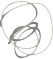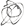Xujiajun Blog

# 31 Jan 2022 Go切片与技巧（附图解）## 2 数组

slice类型是建立在 Go 的数组类型之上的抽象，因此要理解 slice，我们必须首先理解数组。

``````	var a int // 数组类型: 定义指定长度和元素类型
a = 1     // 数组a的第一个索引的值设置为1
i := a
fmt.Println(i)    // 输出：1， 说明已经设置成功
fmt.Println(a) // 输出：0，说明数组不需要显式初始化，其元素本身为零
fmt.Println(a) // 报错：invalid array index 4 (out of bounds for 4-element array)
``````

• 1、代码中 `var a int` 完成了数组的初始化，表示一个由四个整数组成的数组。
• 2、默认值为0。当我们打印`a`的时候输出0。
• 3、设置数组索引的值，可以直接指定下标的索引进行赋值，例子中是`a = 1`
• 3、Go数组的索引和其他语言一样，从0开始，数组的大小是固定的，上面例子中最大是4的的大小，所以下标索引最大是3，所以当我们访问`a`会报数组越界的错误。

`Go的数组是值类型`。不像C语言数组变量是指向第一个元素的指针，所以当我们把数组变量传递或者赋值的时候，其实是做copy的操作。比如下面的例子a赋值给b，修改a中的元素，并没有影响b中的元素：

``````	a := string{"johnny", "太白技术"} // 长度`2`，可以改成`...`，编译器会自动计算数组的长度
b := a
a = "xujiajun"
fmt.Println(a) // 输出：[xujiajun 太白技术]
fmt.Println(b) // 输出：[johnny 太白技术]
``````

``````func double(arr *int) {
for i, num := range *arr {
(*arr)[i] = num * 2
}
}
a := int{1, 2, 3}
double(&a)
fmt.Println(a) // [2 4 6]
``````

（代码参考 Effective Go

## 3 切片的创建

``````type slice struct {
array unsafe.Pointer // 指针指向底层数组
len   int  // 切片长度
cap   int  // 底层数组容量
}
``````

#### 1、变量声明

``````	var s []byte
``````

``````	var x []byte
fmt.Println(cap(x))   // 0
fmt.Println(len(x))   // 0
fmt.Println(x == nil) // true
``````

#### 2、切片字面量

``````a := []string{"johnny", "太白技术"} // 这是切片声明，少了长度的指定
b := string{"johnny", "太白技术"} // 这是数组声明，多了长度的指定
``````

#### 3、make创建：

``````	y := make([]int, 0)
fmt.Println(cap(y))   // 0
fmt.Println(len(y))   // 0
fmt.Println(y == nil) // false
``````

``````	var s []byte
s = make([]byte, 5, 5) // 指定了长度和容量
fmt.Println(s) // 输出：[0 0 0 0 0]

``````

``````s := make([]byte, 5)
fmt.Println(s) // 输出也是 [0 0 0 0 0]
``````

``````	fmt.Println(len(s)) // 5
fmt.Println(cap(s)) // 5
``````

#### 4、使用new

``````	n := *new([]int)
fmt.Println(n == nil) // true
``````

#### 5、从切片/数组截取

``````	n := int{1, 2, 3, 4, 5}
n1 := n[1:]     // 从n数组中截取
fmt.Println(n1) // [2 3 4 5]
n2 := n1[1:]    // 从n1切片中截取
fmt.Println(n2) // [3 4 5]
``````

``````	n2 = 6 // 修改n2，会影响原切片和数组
fmt.Println(n1) // [2 3 6 5]
fmt.Println(n2) // [3 6 5]
fmt.Println(n)  // [1 2 3 6 5]
``````

## 4 切片操作

``````	n := make([]int, 0)
n = append(n, 1)                 // 添加一个元素
n = append(n, 2, 3, 4)           // 添加多个元素
n = append(n, []int{5, 6, 7}...) // 添加一个切片
fmt.Println(n)                   // [1 2 3 4 5 6 7]
``````

``````	fmt.Println(cap(n)) // 容量等于8
n = append(n, []int{8, 9, 10}...)
fmt.Println(cap(n)) // 容量等于16，发生了扩容
``````

## 5 切片表达式

Go语言提供了两种切片的表达式：

• 1、简单表达式
• 2、扩展表达式

### 1、简单的表达式

``````	n := []int{1, 2, 3, 4, 5, 6}
fmt.Println(n[1:4])      // [2 3 4]
``````

``````n := []int{1, 2, 3, 4, 5, 6}
fmt.Println(n[:4])      // [1 2 3 4]，:前面没有值，默认表示0
fmt.Println(n[1:])       // [2 3 4 5 6]，:后面没有值，默认表示切片的长度
``````

#### 边界问题

• 1、当n为数组或字符串表达式n[low:high]中low和high的取值关系：

0 <= low <=high <= len(n)

• 2、当n为切片的时候，表达式n[low:high]中high最大值变成了cap(n)，low和high的取值关系：

0 <= low <=high <= cap(n)

#### 截取字符串

``````	s := "hello taibai"
s1 := s[6:]
fmt.Println(s1)                 // taibai
fmt.Println(reflect.TypeOf(s1)) // string
``````

### 2、扩展表达式

``````	n := []int{1, 2, 3, 4, 5, 6}
n1 := n[1:4]
fmt.Println(n)       // [1 2 3 4 5 6]
fmt.Println(n1)      // [2 3 4]
n1 = append(n1, 100) // 把n的索引位置4的值从原来的5变成了100
fmt.Println(n)       // [1 2 3 4 100 6]
``````

Go 1.2中提供了一种可以限制新切片容量的表达式：

n[low:high:max]

max表示新生成切片的容量，新切片容量等于`max-low`，表达式中low、high、max关系：

0 <= low <= high <= max <= cap(n)

``````	fmt.Println(cap(n[1:4])) // 5
fmt.Println(cap(n[1:4:5])) // 4
``````

#### 关于容量

n[1:4]的长度是3好理解（4-1），容量为什么是5？

``````	n2 := n[1:4:5]         // 长度等于3，容量等于4
fmt.Printf("%p\n", n2) // 0xc0000ac068
n2 = append(n2, 5)
fmt.Printf("%p\n", n2) // 0xc0000ac068
n2 = append(n2, 6)
fmt.Printf("%p\n", n2) // 地址发生改变，0xc0000b8000
``````

## 6 切片技巧

Go在Github的官方Wiki上介绍了切片的技巧SliceTricks，另外这个项目Go Slice Tricks Cheat Sheet基于SliceTricks做了一系列的图，比较直观。Go Slice Tricks Cheat Sheet 做的图有些缺失，我也做了补充

#### AppendVector

``````a = append(a, b...)
``````

#### Copy

``````b := make([]T, len(a))
copy(b, a)

// 效率一般比上面的写法慢，但是如果有更多，他们效率更好
b = append([]T(nil), a...)
b = append(a[:0:0], a...)

// 这个实现等价于make+copy。
// 但在Go工具链v1.16上实际上会比较慢。
b = append(make([]T, 0, len(a)), a...)
``````

### Cut

``````a = append(a[:i], a[j:]...)
``````

### Cut（GC）

``````
copy(a[i:], a[j:])
for k, n := len(a)-j+i, len(a); k < n; k++ {
a[k] = nil // or the zero value of T
}
a = a[:len(a)-j+i]
``````

### Delete

``````a = append(a[:i], a[i+1:]...)
// or
a = a[:i+copy(a[i:], a[i+1:])]
``````

### Delete（GC）

``````copy(a[i:], a[i+1:])
a[len(a)-1] = nil // or the zero value of T
a = a[:len(a)-1]
``````

### Delete without preserving order

``````a[i] = a[len(a)-1]
a = a[:len(a)-1]
``````

### Delete without preserving order（GC）

``````a[i] = a[len(a)-1]
a[len(a)-1] = nil
a = a[:len(a)-1]
``````

### Expand

``````a = append(a[:i], append(make([]T, j), a[i:]...)...)

``````

### Extend

``````a = append(a, make([]T, j)...)
``````

### Filter (in place)

``````n := 0
for _, x := range a {
if keep(x) {
a[n] = x
n++
}
}
a = a[:n]
``````

### Insert

``````a = append(a[:i], append([]T{x}, a[i:]...)...)
``````

``````s = append(s, 0 /* 先添加一个0值*/)
copy(s[i+1:], s[i:])
s[i] = x
``````

### InsertVector

``````a = append(a[:i], append(b, a[i:]...)...)
``````
``````func Insert(s []int, k int, vs ...int) []int {
if n := len(s) + len(vs); n <= cap(s) {
s2 := s[:n]
copy(s2[k+len(vs):], s[k:])
copy(s2[k:], vs)
return s2
}
s2 := make([]int, len(s) + len(vs))
copy(s2, s[:k])
copy(s2[k:], vs)
copy(s2[k+len(vs):], s[k:])
return s2
}

a = Insert(a, i, b...)
``````

### Push

``````a = append(a, x)
``````

### Pop

`````` x, a = a[len(a)-1], a[:len(a)-1]
``````

### Push Front/Unshift

``````a = append([]T{x}, a...)
``````

### Pop Front/Shift

``````x, a = a, a[1:]

``````

## 7 切片额外技巧

### Filtering without allocating

``````b := a[:0]
for _, x := range a {
if f(x) {
b = append(b, x)
}
}
``````

``````for i := len(b); i < len(a); i++ {
a[i] = nil // or the zero value of T
}
``````

### Reversing

``````for i := len(a)/2-1; i >= 0; i-- {
opp := len(a)-1-i
a[i], a[opp] = a[opp], a[i]
}
``````

``````for left, right := 0, len(a)-1; left < right; left, right = left+1, right-1 {
a[left], a[right] = a[right], a[left]
}
``````

### Shuffling

``````for i := len(a) - 1; i > 0; i-- {
j := rand.Intn(i + 1)
a[i], a[j] = a[j], a[i]
}
``````

go1.10之后有内置函数Shuffle

### Batching with minimal allocation

``````actions := []int{0, 1, 2, 3, 4, 5, 6, 7, 8, 9}
batchSize := 3
batches := make([][]int, 0, (len(actions) + batchSize - 1) / batchSize)

for batchSize < len(actions) {
actions, batches = actions[batchSize:], append(batches, actions[0:batchSize:batchSize])
}
batches = append(batches, actions)

// 结果：
// [[0 1 2] [3 4 5] [6 7 8] ]

``````

### In-place deduplicate (comparable)

``````import "sort"

in := []int{3,2,1,4,3,2,1,4,1} // any item can be sorted
sort.Ints(in)
j := 0
for i := 1; i < len(in); i++ {
if in[j] == in[i] {
continue
}
j++
// preserve the original data
// in[i], in[j] = in[j], in[i]
// only set what is required
in[j] = in[i]
}
result := in[:j+1]
fmt.Println(result) // [1 2 3 4]
``````

### Move to front, or prepend if not present, in place if possible.

``````// moveToFront moves needle to the front of haystack, in place if possible.
func moveToFront(needle string, haystack []string) []string {
if len(haystack) != 0 && haystack == needle {
return haystack
}
prev := needle
for i, elem := range haystack {
switch {
case i == 0:
haystack = needle
prev = elem
case elem == needle:
haystack[i] = prev
return haystack
default:
haystack[i] = prev
prev = elem
}
}
return append(haystack, prev)
}

haystack := []string{"a", "b", "c", "d", "e"} // [a b c d e]
haystack = moveToFront("c", haystack)         // [c a b d e]
haystack = moveToFront("f", haystack)         // [f c a b d e]
``````

### Sliding Window

``````func slidingWindow(size int, input []int) [][]int {
// returns the input slice as the first element
if len(input) <= size {
return [][]int{input}
}

// allocate slice at the precise size we need
r := make([][]int, 0, len(input)-size+1)

for i, j := 0, size; j <= len(input); i, j = i+1, j+1 {
r = append(r, input[i:j])
}

return r
}

func TestSlidingWindow(t *testing.T) {
result := slidingWindow(2, []int{1, 2, 3, 4, 5})
fmt.Println(result) // [[1 2] [2 3] [3 4] [4 5]]
}
``````

## 总结

• 1、Go切片本质上是一个结构体，保存了其长度、底层数组的容量、底层数组的指针。
• 2、Go切片创建方式比较多样：`变量声明``切片字面量``make创建``new创建``从切片/数组截取`
• 3、Go切片使用`len()`计算长度、`cap()`计算容量、`append()`来添加元素。
• 4、Go切片相比数组更灵活，有很多技巧，也正因为灵活，容易发生类似内存泄露的问题，需要注意。

Til next time,
Xujiajun at 00:00